# Coordinates

Determine the coordinates of the vertices and the content of the parallelogram, the two sides of which lie on the lines 8x + 3y + 1 = 0, 2x + y-1 = 0 and the diagonal on the line 3x + 2y + 3 = 0

Correct result:

x0 =  -2
y0 =  5
x1 =  1
y1 =  -3
x2 =  5
y2 =  -9
x3 =  8
y3 =  -17
S =  14

#### Solution:

8x0+3y0+1=0
2x0+y0-1=0

8•x0+3•y0+1=0
2•x0+y0-1=0

8x0+3y0 = -1
2x0+y0 = 1

x0 = -2
y0 = 5

Our linear equations calculator calculates it.

8x1+3y1+1=0
3x1+ 2y1+3=0

8•x1+3•y1+1=0
3•x1+ 2•y1+3=0

8x1+3y1 = -1
3x1+2y1 = -3

x1 = 1
y1 = -3

Our linear equations calculator calculates it.

2x2+y2-1=0
3x2+ 2y2+3=0

2•x2+y2-1=0
3•x2+ 2•y2+3=0

2x2+y2 = 1
3x2+2y2 = -3

x2 = 5
y2 = -9

Our linear equations calculator calculates it.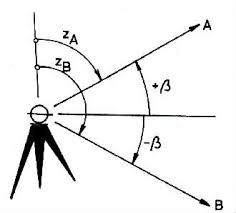We would be pleased if you find an error in the word problem, spelling mistakes, or inaccuracies and send it to us. Thank you!Tips to related online calculators
For Basic calculations in analytic geometry is a helpful line slope calculator. From coordinates of two points in the plane it calculate slope, normal and parametric line equation(s), slope, directional angle, direction vector, the length of segment, intersections the coordinate axes etc.
Do you have a system of equations and looking for calculator system of linear equations?

#### You need to know the following knowledge to solve this word math problem:

We encourage you to watch this tutorial video on this math problem:

## Next similar math problems:

• Diamond area from diagonalsIn the diamond ABCD is AB = 4 dm and the length of the diagonal is 6.4 dm long. What is the area of the diamond?
• Garden exchangeThe garden has the shape of a rectangular trapezoid, the bases of which have dimensions of 60 m and 30 m and a vertical arm of 40 m. The owner exchanged this garden for a parallelogram, the area of which is 7/9 of the area of a trapezoidal garden. What is
• Children playgroundThe playground has the shape of a trapezoid, the parallel sides have a length of 36 m and 21 m, the remaining two sides are 14 m long and 16 m long. Determine the size of the inner trapezoid angles.
• Parallelogram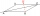Rhomboid (parallelogram) has a longer side of 50 cm long. The size of its one height is four times the size of its second height. Calculate the length of the shorter side of this rhomboid in the centimeters.
• Parallelogram perimeter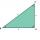The ABC triangle with sides a = 5cm, b = 3cm, c = 40mm has the center of the sides of the K,L,M. How many cm have the KBLM parallelogram perimeter?
• Parallelogram +ľ| AB | = 76cm, | BC | = 44cm, angle BAD = 30 °. Find the area of the parallelogram.Show that the quadrilateral with vertices P1(0,1), P2(4,2) P3(3,6) P4(-5,4) has two right triangles.
• ParallelogramCalculate the missing side of a parallelogram, if you know the perimeter o and one side: a) o = 7.2 cm; b = 1.8 cm b) o = 5.4 cm; a = 1.9 cm
• MidpointsTriangle ABC with sides a = 5 cm, b = 3 cm, c = 40mm has a midpoint of K, L, M. How many centimeters is long perimeter of parallelogram KBLM?
• Trapezoid MO-5-Z8ABCD is a trapezoid that lime segment CE is divided into a triangle and parallelogram, as shown. Point F is the midpoint of CE, DF line passes through the center of the segment BE, and the area of the triangle CDE is 3 cm2. Determine the area of the trape
• Parallelogram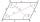In the parallelogram we know one internal angle 67°33`. Calculate the other internal angles.
• Rectangle - parallelogram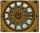It is given a rectangle that is circumscribed by a circle with a radius of 5 cm. The short side of the rectangle measures 6 cm. Calculate the perimeter of a parallelogram ABCD whose vertices are the midpoints of the sides of the rectangle.
• PrismThe volume of tetrahedral prism is 2.43 m3. Base of prism is a parallelogram in which a side 2,5dm and height ha = 18cm. Calculate the height of the prism.
• Colour - billboardShelftalker has the shape of a parallelogram. Its length is 4.9 m and the corresponding height is 3.5 meters. Calculate how much (kg) paint must purchase to redecoration if 1 kg cover 4 m2 of shelftalker surface.
• The farmer field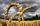The field has paralleogram shape with dimensions side a = 80 m and height 296 m. Two and two sides is at angle 80°. Calculate acreage of field in hectares.
• ParallelogramThe parallelogram has sides lengths in the ratio 3: 4 and perimeter 2.8 meters. Determine the lengths of the sides.
• Parallelogram ABCDThe area of parallelogram ABCD is 440 cm2. Points M and N are the midpoints of the sides AB and BC. What is the area of a quadrilateral MBND?